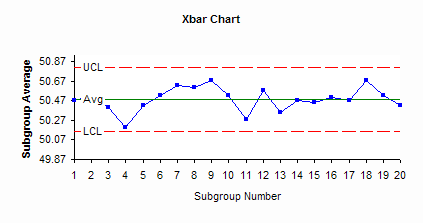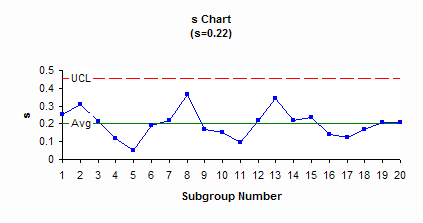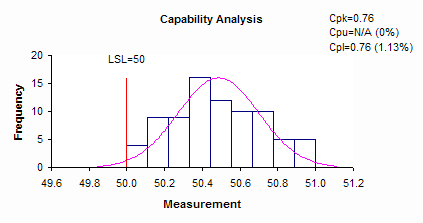October 2008

In this Issue:

This month is the second publication on X-s charts. Last month we introduced the chart and provided the steps in constructing an X-s chart. This month, we will look at a detailed example of an X-s chart. The X-s chart is a type of control chart that can be used with variables data. Like most other variables control charts, it is actually two charts. One chart is for subgroup averages (X). The other chart is for subgroup standard deviations (s). These charts are a very powerful tool for monitoring variation in a process and detecting changes in either the average or the amount of variation in the process.

### Bag Weight Process

A process involves the filling of bags with sand. Each bag is supposed to weigh a minimum of 50 pounds. The process has an automatic method of weighing the bags. Once the bag reaches 50 pounds, the filling operation is supposed to stop. To determine how much variation there is in the bag weights, an operator takes the first four bags filled each hour and manually weighs them using a calibrated scale. The data is shown in the table below.

 Subgroup Number (k) 1 2 3 4 1 50.4 50.2 50.5 50.8 2 50.7 50.8 50.1 50.5 3 50.7 50.3 50.4 50.2 4 50.1 50.3 50.3 50.1 5 50.4 50.4 50.5 50.4 6 50.4 50.4 50.8 50.5 7 50.5 50.4 50.9 50.7 8 51 50.4 50.8 50.2 9 50.6 50.7 50.9 50.5 10 50.4 50.6 50.4 50.7 11 50.2 50.3 50.2 50.4 12 50.5 50.5 50.4 50.9 13 50.2 50.4 50 50.8 14 50.2 50.4 50.7 50.6 15 50.7 50.6 50.2 50.3 16 50.6 50.6 50.3 50.5 17 50.5 50.6 50.5 50.3 18 50.9 50.6 50.5 50.7 19 50.7 50.4 50.3 50.7 20 50.3 50.2 50.6 50.6

We want to use an X-s chart to find out if this process is consistent and predictable (in control) as well as if it is capable of producing bag weights with a minimum of 50 pounds.

The calculations needed to construct the X-s chart were covered in last month's newsletter. For practice, you can enter the data from this newsletter into Excel and see if you can get the same results as shown below. The subgroup size is 4. For the data above, the following averages and control limits can be generated.

For the X chart:

• Average = 50.49
• UCL = 50.81
• LCL = 50.16

For the standard deviation chart:

• Average = 0.2
• UCL = .45
• LCL = None

### X-s Charts for the Bag Weights

The X-s charts are shown below. Are these charts in control? For information on interpreting control charts, please see our past publications. Interpreting control charts was covered in April 2004.### Interpreting the Charts

The X  and s charts above are in statistical control. This means that the process is consistent and predictable. There are only common causes of variation present (see the January 2004 publication on variation for more information on common and special causes of variation). What does it mean for an s chart to be in statistical control? It means that variation within the subgroup is consistent from subgroup to subgroup. For the bag weight example, it means that the standard deviation of each subgroup is the "same" for all subgroups. There is no statistical difference between these standard deviations. The average standard deviation will be 0.2 pounds but it can vary anywhere from 0 to 0.45 pounds. Since the s chart is in control, you can estimate the standard deviation of the individual values using the formula provided in last month's publication The standard deviation is 0.22.

What does it mean for the X chart to be in statistical control? It means that the variation between subgroup averages is the same from subgroup to subgroup. For the bag weight example, it means that there is no statistical difference between the subgroup averages. As long as the process stays the same, we can predict the average bag weights for the four samples. The long-term average will be 50.49. The average will vary from 50.16 to 50.81.

### Is the Process Capable?

Can the process meet the specification of having a minimum bag weight of 50? Be careful here. The X chart plots the subgroup averages. The LCL on the X chart is 50.16. This means that the average of the four bag weights will not be below 50.16 as long as the process stays the same. However, the specification deals with individual bag weights, not averages. Individual values will vary more than the subgroup averages. Just because you do not have any subgroup averages below 50, does not mean that you will not have any individual bag weights below 50.

Information on process capability is given on our website. We have a three part series on process capability. The process capability chart for the bag weight process is shown below. What can you conclude about the process capability?Note that the data in the table above do not have any bag weights below 50. However, the process capability analysis shows that the Cpk = 0.76. Since this is less than 1, it means that there is out-of-specification material - some bags will weigh less than 50 pounds. The analysis predicts about 1% of the bags will weigh less than 50 pounds. The only way to prevent any underweight bags from going to the customer with the current process is to inspect 100% of the bags. Of course, the best approach would be to improve the process - to move the average higher or reduce the amount of variation - so no bags will be produced that weigh less than 50 pounds.

### Comparison to X-R Charts

There is very little difference in the conclusions you reach if you use an X-R chart or an X-s chart. The control limits on the X chart will essentially be the same. In 2005, we had a multi-part series on X-R charts. If you look at the April 2005 newsletter, you will see the same bag weight example. The results are essentially identical.

### Summary

This month’s publication continued our look at X-s charts.  A detailed example was covered.  The X-s chart was interpreted and explained.   Being in control does not mean being capable.  In this example, the processs was not capable.  The X-s and X-R charts give very similar results.

SPC for Excel Software

SPC Training

SPC Consulting

Ordering Information

Thanks so much for reading our publication. We hope you find it informative and useful. Happy charting and may the data always support your position.

Sincerely,

Dr. Bill McNeese
BPI Consulting, LLC